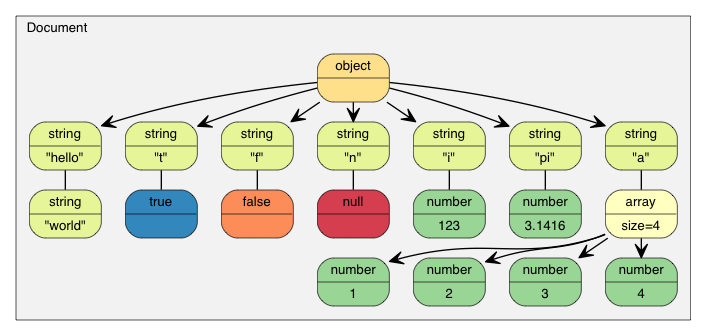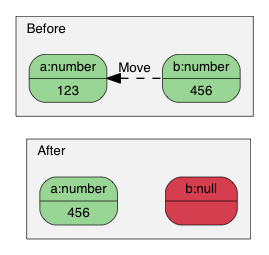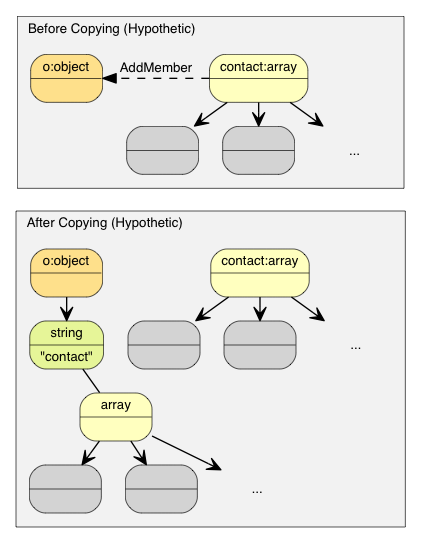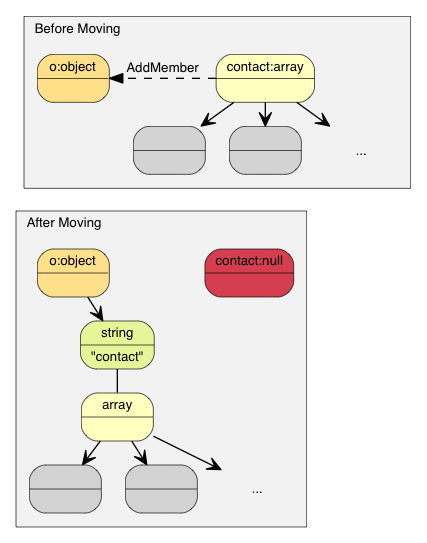# 查询 Value

```{
"hello": "world",
"t": true ,
"f": false,
"n": null,
"i": 123,
"pi": 3.1416,
"a": [1, 2, 3, 4]
}
```

```#include "rapidjson/document.h"

using namespace rapidjson;

// ...
Document document;
document.Parse(json);
``````assert(document.IsObject());
```

```assert(document.HasMember("hello"));
assert(document["hello"].IsString());
printf("hello = %s\n", document["hello"].GetString());
```
```world
```

JSON True/False 值是以 `bool` 表示的。

```assert(document["t"].IsBool());
printf("t = %s\n", document["t"].GetBool() ? "true" : "false");
```
```true
```

JSON Null 值可用 `IsNull()` 查询。

```printf("n = %s\n", document["n"].IsNull() ? "null" : "?");
```
```null
```

JSON Number 类型表示所有数值。然而，C++ 需要使用更专门的类型。

```assert(document["i"].IsNumber());

// 在此情况下，IsUint()/IsInt64()/IsUint64() 也会返回 true
assert(document["i"].IsInt());
printf("i = %d\n", document["i"].GetInt());
// 另一种用法： (int)document["i"]

assert(document["pi"].IsNumber());
assert(document["pi"].IsDouble());
printf("pi = %g\n", document["pi"].GetDouble());
```
```i = 123
pi = 3.1416
```

JSON Array 包含一些元素。

```// 使用引用来连续访问，方便之余还更高效。
const Value& a = document["a"];
assert(a.IsArray());
for (SizeType i = 0; i < a.Size(); i++) // 使用 SizeType 而不是 size_t
printf("a[%d] = %d\n", i, a[i].GetInt());
```
```a = 1
a = 2
a = 3
a = 4
```

## 查询 Array

Array 与 `std::vector` 相似，除了使用索引，也可使用迭代器来访问所有元素。

```for (Value::ConstValueIterator itr = a.Begin(); itr != a.End(); ++itr)
printf("%d ", itr->GetInt());
```

• `SizeType Capacity() const`
• `bool Empty() const`

### 范围 for 循环 (v1.1.0 中的新功能)

```for (auto& v : a.GetArray())
printf("%d ", v.GetInt());
```

## 查询 Object

```static const char* kTypeNames[] =
{ "Null", "False", "True", "Object", "Array", "String", "Number" };

for (Value::ConstMemberIterator itr = document.MemberBegin();
itr != document.MemberEnd(); ++itr)
{
printf("Type of member %s is %s\n",
itr->name.GetString(), kTypeNames[itr->value.GetType()]);
}
```
```Type of member hello is String
Type of member t is True
Type of member f is False
Type of member n is Null
Type of member i is Number
Type of member pi is Number
Type of member a is Array
```

```Value::ConstMemberIterator itr = document.FindMember("hello");
if (itr != document.MemberEnd())
printf("%s\n", itr->value.GetString());
```

### 范围 for 循环 (v1.1.0 中的新功能)

```for (auto& m : document.GetObject())
printf("Type of member %s is %s\n",
m.name.GetString(), kTypeNames[m.value.GetType()]);
```

## 查询 Number

JSON 只提供一种数值类型──Number。数字可以是整数或实数。RFC 4627 规定数字的范围由解析器指定。

`unsigned`32 位无号整数
`int`32 位有号整数
`uint64_t`64 位无号整数
`int64_t`64 位有号整数
`double`64 位双精度浮点数

`bool IsNumber()`不适用
`bool IsUint()``unsigned GetUint()`
`bool IsInt()``int GetInt()`
`bool IsUint64()``uint64_t GetUint64()`
`bool IsInt64()``int64_t GetInt64()`
`bool IsDouble()``double GetDouble()`

## 查询 String

```{ "s" :  "a\u0000b" }
```

`"a\u0000b"` 值的正确长度应该是 3。但 `strlen()` 会返回 1。

`GetStringLength()` 也可以提高性能，因为用户可能需要调用 `strlen()` 去分配缓冲。

```string(const char* s, size_t count);
```

## 比较两个 Value

```if (document["hello"] == document["n"]) /*...*/;    // 比较两个值
if (document["hello"] == "world") /*...*/;          // 与字符串字面量作比较
if (document["i"] != 123) /*...*/;                  // 与整数作比较
if (document["pi"] != 3.14) /*...*/;                // 与 double 作比较
```

Array／Object 顺序以它们的元素／成员作比较。当且仅当它们的整个子树相等，它们才当作相等。

# 创建／修改值

## 改变 Value 类型

```Document d; // Null
d.SetObject();

Value v;    // Null
v.SetInt(10);
v = 10;     // 简写，和上面的相同
```

### 构造函数的各个重载

```Value b(true);    // 调用 Value(bool)
Value i(-123);    // 调用 Value(int)
Value u(123u);    // 调用 Value(unsigned)
Value d(1.5);     // 调用 Value(double)
```

```Value o(kObjectType);
Value a(kArrayType);
```

## 转移语义（Move Semantics）

```Value a(123);
Value b(456);
b = a;         // a 变成 Null，b 变成数字 123。
``````Value o(kObjectType);
{
Value contacts(kArrayType);
// 把元素加进 contacts 数组。
// ...
o.AddMember("contacts", contacts, d.GetAllocator());  // 深度复制 contacts （可能有大量内存分配）
// 析构 contacts。
}
``````Value o(kObjectType);
{
Value contacts(kArrayType);
// adding elements to contacts array.
o.AddMember("contacts", contacts, d.GetAllocator());  // 只需 memcpy() contacts 本身至新成员的 Value（16 字节）
// contacts 在这里变成 Null。它的析构是平凡的。
}
```### 转移语义及临时值

```Value a(kArrayType);
Document::AllocatorType& allocator = document.GetAllocator();
// a.PushBack(Value(42), allocator);       // 不能通过编译
a.PushBack(Value().SetInt(42), allocator); // fluent API
a.PushBack(Value(42).Move(), allocator);   // 和上一行相同
```

## 创建 String

RapidJSON 提供两个 String 的存储策略。

1. copy-string: 分配缓冲区，然后把来源数据复制至它。
2. const-string: 简单地储存字符串的指针。

Copy-string 总是安全的，因为它拥有数据的克隆。Const-string 可用于存储字符串字面量，以及用于在 DOM 一节中将会提到的 in-situ 解析中。

```Document document;
Value author;
char buffer;
int len = sprintf(buffer, "%s %s", "Milo", "Yip"); // 动态创建的字符串。
author.SetString(buffer, len, document.GetAllocator());
memset(buffer, 0, sizeof(buffer));
// 清空 buffer 后 author.GetString() 仍然包含 "Milo Yip"
```

```Value s;
s.SetString("rapidjson");    // 可包含空字符，长度在编译期推导
s = "rapidjson";             // 上行的缩写
```

```const char * cstr = getenv("USER");
size_t cstr_len = ...;                 // 如果有长度
Value s;
// s.SetString(cstr);                  // 这不能通过编译
s.SetString(StringRef(cstr));          // 可以，假设它的生命周期安全，并且是以空字符结尾的
s = StringRef(cstr);                   // 上行的缩写
s.SetString(StringRef(cstr, cstr_len));// 更快，可处理空字符
s = StringRef(cstr, cstr_len);         // 上行的缩写

```

## 修改 Array

Array 类型的 Value 提供与 `std::vector` 相似的 API。

• `Clear()`
• `Reserve(SizeType, Allocator&)`
• `Value& PushBack(Value&, Allocator&)`
• `template <typename T> GenericValue& PushBack(T, Allocator&)`
• `Value& PopBack()`
• `ValueIterator Erase(ConstValueIterator pos)`
• `ValueIterator Erase(ConstValueIterator first, ConstValueIterator last)`

```Value a(kArrayType);
Document::AllocatorType& allocator = document.GetAllocator();

for (int i = 5; i <= 10; i++)
a.PushBack(i, allocator);   // 可能需要调用 realloc() 所以需要 allocator

// 流畅接口（Fluent interface）
a.PushBack("Lua", allocator).PushBack("Mio", allocator);
```

```// 就地 Value 参数
contact.PushBack(Value("copy", document.GetAllocator()).Move(), // copy string
document.GetAllocator());

// 显式 Value 参数
Value val("key", document.GetAllocator()); // copy string
contact.PushBack(val, document.GetAllocator());
```

## 修改 Object

Object 是键值对的集合。每个键必须为 String。要修改 Object，方法是增加或移除成员。以下的 API 用来增加成员：

• `Value& AddMember(Value&, Value&, Allocator& allocator)`
• `Value& AddMember(StringRefType, Value&, Allocator&)`
• `template <typename T> Value& AddMember(StringRefType, T value, Allocator&)`

```Value contact(kObject);
```

```// 就地 Value 参数
Value().Move(),                                // null value
document.GetAllocator());

// 显式参数
Value key("key", document.GetAllocator()); // copy string name
Value val(42);                             // 某 Value
```

• `bool RemoveMember(const Ch* name)`：使用键名来移除成员（线性时间复杂度）。
• `bool RemoveMember(const Value& name)`：除了 `name` 是一个 Value，和上一行相同。
• `MemberIterator RemoveMember(MemberIterator)`：使用迭代器移除成员（_ 常数 _ 时间复杂度）。
• `MemberIterator EraseMember(MemberIterator)`：和上行相似但维持成员次序（线性时间复杂度）。
• `MemberIterator EraseMember(MemberIterator first, MemberIterator last)`：移除一个范围内的成员，维持次序（线性时间复杂度）。

`MemberIterator RemoveMember(MemberIterator)` 使用了“转移最后”手法来达成常数时间复杂度。基本上就是析构迭代器位置的成员，然后把最后的成员转移至迭代器位置。因此，成员的次序会被改变。

## 深复制 Value

```Document d;
Document::AllocatorType& a = d.GetAllocator();
Value v1("foo");
// Value v2(v1); // 不容许

Value v2(v1, a);                      // 制造一个克隆
assert(v1.IsString());                // v1 不变
d.SetArray().PushBack(v1, a).PushBack(v2, a);
assert(v1.IsNull() && v2.IsNull());   // 两个都转移动 d

v2.CopyFrom(d, a);                    // 把整个 document 复制至 v2
assert(d.IsArray() && d.Size() == 2); // d 不变
d.PushBack(v1, a);
```

## 交换 Value

RapidJSON 也提供 `Swap()`

```Value a(123);
Value b("Hello");
a.Swap(b);
assert(a.IsString());
assert(b.IsInt());
```

# 下一部分

1. 是读写 JSON 的通道。流可以是内存字符串、文件流等。用户也可以自定义流。
2. 编码 定义在流或内存中使用的字符编码。RapidJSON 也在内部提供 Unicode 转换及校验功能。
3. DOM 的基本功能已在本教程里介绍。还有更高级的功能，如原位（in situ）解析、其他解析选项及高级用法。
4. SAX 是 RapidJSON 解析／生成功能的基础。学习使用 `Reader`/`Writer` 去实现更高性能的应用程序。也可以使用 `PrettyWriter` 去格式化 JSON。
5. 性能 展示一些我们做的及第三方的性能测试。
6. 技术内幕 讲述一些 RapidJSON 内部的设计及技术。